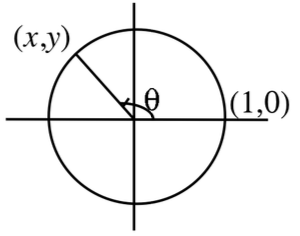### Home > PC > Chapter 10 > Lesson 10.2.1 > Problem10-71

10-71.

In parametric equations, the parameter, or “helping variable,” does not have to be time. In fact, you have studied parametric equations extensively in Chapter 4 without knowing it!

1. Use the diagram to express $x$ in terms of $θ$.

$\cos \theta=\frac{\textit{x}}{1}$

2. Use the diagram to express $y$ in terms of $θ$.

$\sin \theta=\frac{\textit{y}}{1}$

3. From parts (a) and (b) we know that the parametric equations for the unit circle are $x = \cos θ$ and $y = \sin θ$. Make a table for $\theta = 0 , \frac { \pi } { 3 } , \frac { \pi } { 2 } , \frac { 2 \pi } { 3 } , \pi$etc. up to $3\pi$, with three columns using $θ$, $x$, and $y$.

4. Plot the $x$- and $y$-values you generated on graph paper to see the circle.

5. Write parametric equations for the circle of radius $5$ centered at the origin.

$\cos \theta=\frac{\textit{x}}{5}$

6. Write parametric equations for the circle of radius $5$ centered at $\left(7, 9\right)$.

$\sin \theta=\frac{\textit{y}-9}{5}$